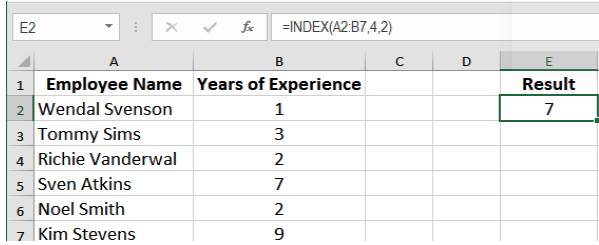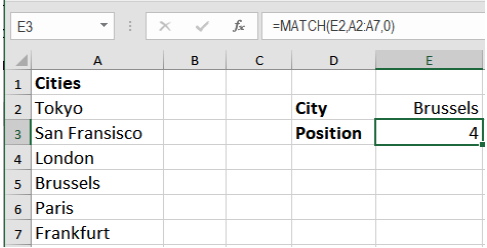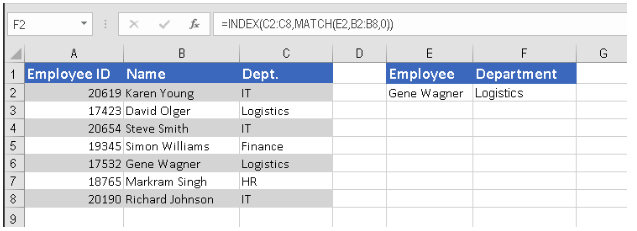Get instant live expert help with Excel or Google Sheets“My Excelchat expert helped me in less than 20 minutes, saving me what would have been 5 hours of work!”

#### Post your problem and you’ll get expert help in seconds.

Your message must be at least 40 characters
Our professional experts are available now. Your privacy is guaranteed.

# How to Use the INDEX MATCH Function in Excel

INDEX and MATCH are two functions in Excel that can be combined together to perform advanced lookups. It is a powerful duo of functions that generally overcomes all the limitations of the VLOOKUP function, i.e., unlike VLOOKUP, INDEX MATCH does not require the lookup value being in the first column.

This allows you to lookup in the columns left to the lookup value which is not possible with VLOOKUP. INDEX MATCH uses a dynamic reference which leads to fewer errors than a static column reference used by VLOOKUP leading to more errors. VLOOKUP breaks if you insert/delete new columns, whereas INDEX MATCH handles insertion/deletion well because of the dynamic reference.

## How to Use INDEX MATCH Function

To use the INDEX MATCH function in Excel, you have to nest the MATCH function inside the INDEX function. It follows the syntax.`=INDEX(range, MATCH(lookup_value, lookup_range, match_type)).` It is important to realize that INDEX MATCH isn’t actually a standalone function, but rather a combination of Excel’s INDEX and MATCH functions.

### The INDEX Function

The INDEX function in Excel returns a value at a cell from a table or range. Given the row and column numbers, It extracts the values from the table or range. Index follows the structure `=INDEX (array, row_num, [col_num], [area_num]).`In this example, the formula `=INDEX(A2:B7,4,2)` in cell E2 returns a reference to row 4 of the range A2:B7, resulting in cell B5. This contains the entry 7.

### The MATCH Function

The MATCH function in Excel looks up for a value in a table or array and returns the relative position of the lookup value.Assigning the formula` =MATCH(E2, A2:A7, 0)` to cell E3 returns the relative position of the lookup value Brussels which is 4. MATCH performs an exact match here as the match_typeis set to 0.

### How to nest a MATCH function in an INDEX Function

Now that you know the basics, it’s time to show how INDEX and MATCH work together.

The MATCH function determines the relative position of the lookup value in the specified range of cells. From there, the INDEX function takes that number, or numbers, and returns a value in the corresponding cell. Combined together, The INDEX and MATCH functions look like:

`=INDEX(range, MATCH(lookup_value, lookup_range, match_type))`

Let’s take a closer look at what’s going on here. First, you call INDEX on a range of numbers from which you look up the value. Then, MATCH tells Excel how many cells it should count into INDEX’s range, based on a given value matched against a separate array.

Let’s look at an example that has INDEX and MATCH in action. Assign the formula `=INDEX(C2:C8,MATCH(E2,B2:B8,0)) `in cell F2, and it will return the Dept. Logistics for the employee Gene Wagner.Now, let’s analyze what each component of this formula actually does:

• The MATCH function searches for the lookup value Gene Wagner in column B, more precisely cells `B2:B8,` and returns the number 5, because Gene Wagner is the fifth in the list.
• The INDEX functions takes 5 in the second parameter (row_num), which indicates which row you want to return a value from, and turns into a simple `=INDEX(\$C\$2:\$C\$8,5)`. Translated into plain English, the formula reads: search in cells C2 through C8 and return a value of the cell in the 5th row, i.e., cell C6, because you start counting from the second row.

Using INDEX and MATCH is a great way for lookup in Excel. It overcomes the limitations of VLOOKUP and allows calculation with greater efficiency and speed.

Still need some help with Excel formatting or have other questions about Excel? Connect with a live Excel expert here for some 1 on 1 help. Your first session is always free.

### Did this post not answer your question? Get a solution from connecting with the expert.Another blog reader asked this question today on Excelchat:
Solution examplesindex and match with duplicates. I need to use another column as a reference, so my return value has two match the value of two things for it to return
Solved by E. H. in 60 minsI have a file with two sheets. On the 1st, I have dollars in O13and in O16 a number signifying 1-6 people. On the second workbook is a matrix that corresponds to the dollar amount AND the number of people. For example, sheet 1 O13 is \$6,000. O16 is 3. I want a formula to find \$6,000 (not higher) on sheet 2 in column A. Then I want it to compare sheet 1 O16 to the correct column (B-G representing 1-6) on sheet 2 for an exact match to place in sheet1 O17. The range of sheet1 O13 is referenced on sheet2 A5-A383, and sheet 1 O16 is referenced on sheet2 B5:G383. I have an INDEX Match but it isn't quite working, yet.
Solved by B. B. in 60 minsI have two lists. One is a list of part numbers. The other is a list of long text descriptions. I can use VLOOKUP with "*"&cell address"*" to find the first match. But how can I find the second and third matches? I have tried using INDEX and SHORT but the wild cards no longer find the match like they do in VLOOKUP. Can you help?
Solved by S. J. in 36 minsHello, I have a big spreadsheet in which I need to know how many patients came in each month based on provider. I am using the following formula but I still get the N/A error. =INDEX('No Show Appts Data'!D:E, MATCH(1, 'No Show Appts Data'!D:D='No Shows Data'!M2)*('No Shows Data'!E:E='No Shows Data'!N1),0)) No Show Appts Data is the name of the sheet where Column D is Month Year of date in question and Column E is the provider. No Shows Data is the sheet where I am making all the formula calculation where Column M is Month and Year and Column N is the provider in question and therefore M2 is the month in question and N1 the provider in question. How do I fix this error? Thanks
Solved by F. H. in 40 minsI need to find an INDEX function that will convert the Call Day (a number 1-7) to the actual weekday found in row 1 of the DayofWeek named range. I don't know if I'm supposed to use the MATCH function as well or not.
Solved by D. D. in 11 mins## Subscribe to Excelchat.coAnother blog reader asked this question today on Excelchat: